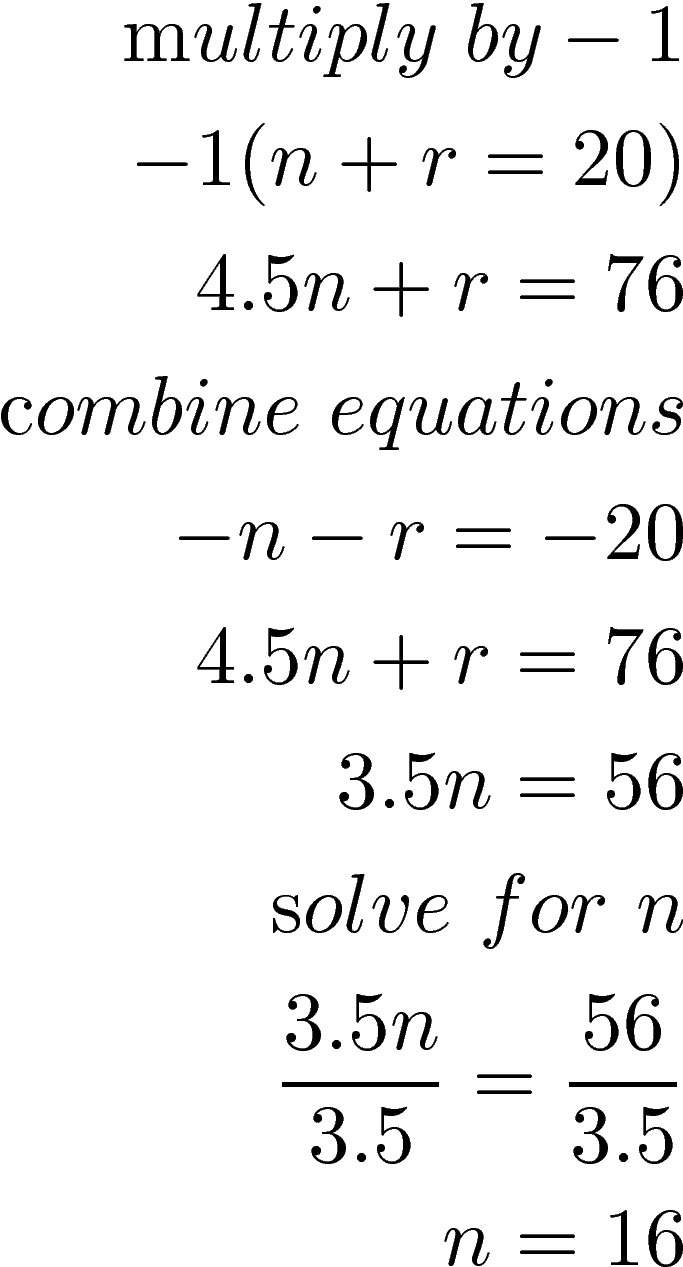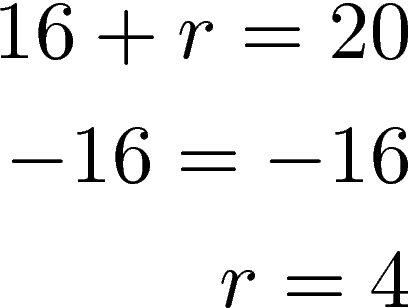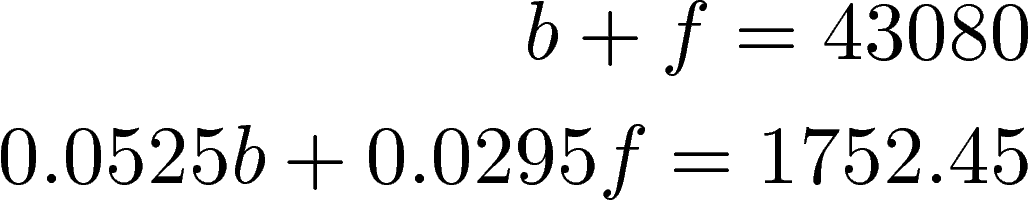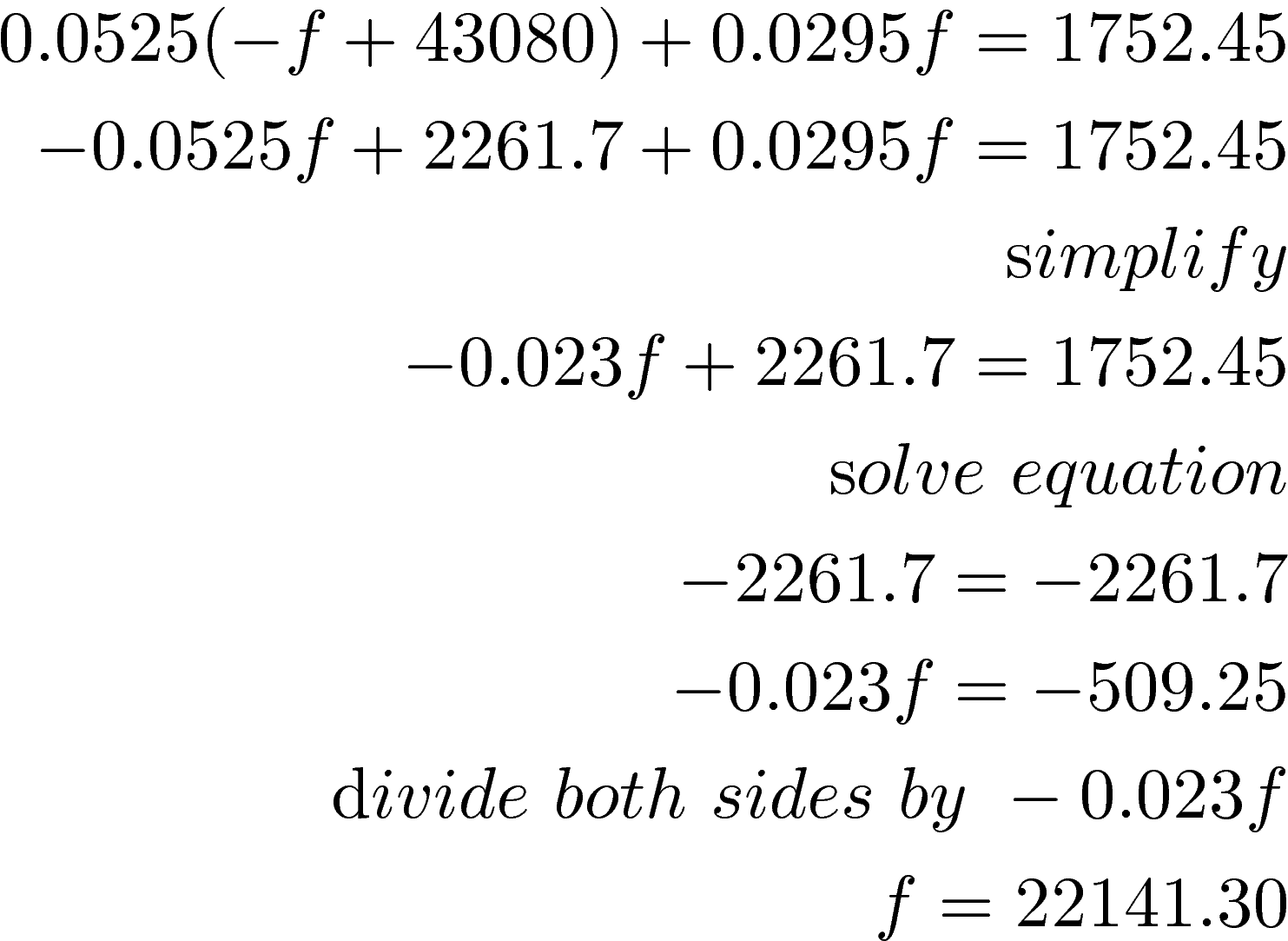# System of Equations and Mixture Application

Solving a system of equations with a mixture application involves combining two or more quantities. The general setup for the equations is as follows

Quantity * value = totalThis equation is used for both equations. You simply read the problem and plug in the information. The examples in this post are primarily related to business as this is one of the more practical applications of solving a system of equations for the average person. However, a system of equations for mixtures can also be used for determining solutions but this is more common in chemistry.

Example 1: Making Food

John wants to make 20 lbs of granola using nuts and raisins. His budget requires that the granola cost \$3.80 per pound. Nuts are \$4.50 per pound and raisins are \$1.00 per pound. How many pounds of nuts and raisins can he use?

The first thing we need to determine what we know

• cost of the raisins
• cost of the nuts
• total cost of the granola
• number of pounds of granola to make

Below is all of our information in a table

Pounds * Price Total
Nuts n 4.50 4.5n
Raisins r 1 r
Granola 20 3.80 3.8(20) = 76

What we need to know is how many pounds of nuts and raisins can we use to have the total price per pound be \$3.80.

With this information, we can set up our system of equations. We take the pounds column and create the first equation and the total column to create the second equation.We will use elimination to solve this system. We will multiply the first equation by -1 and combine them. Then we solve for n as in the steps belowWe know n = 16 or that we can have 16 pounds of nuts. To determine the amount of raisins we use our first equation in the system.You can check this yourself if you desire.

Example 2: Interests

Below is an example that involves two loans with different interest rates. Our job will be to determine the principal amount of the loan.

Tom owes \$43,080 on two student loans. The bank’s interest rate is 5.25% and the federal loan rate is 2.95%. The total amount of interest he paid last two years was 6678.72. What was the principal for each loan

The first thing we need to determine what we know

• bank interest rate
• Federal interest rate
• time of repayment
• Amount of loan
• Interest paid so far

Below is all of our information in a table

Principal * Rate Time Total
Bank b 0.0525 1 0.0525b
Federal f 0.0295 1 0.0295f
Total 43080 1752.45

Below is our system of equationTo solve the system of equations we will use substitution. First, we need to solve for b as shown belowWe now substitute  and solveWe know the federal loan is \$22,141.30 we can use this information to find the bank loan amount using the first equation.The bank loan was \$20,938.70

Conclusion

Hopefully, it is clear by now that solving a system of equations can have real-world significance. Applications of this concept can be useful in the context of business as shown here.